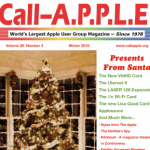# Applesoft’s Input Statement

by Andrew McKellar
Australian Apple Review June 1987

Using Applesoft’s INPUT statement does not allow great control over what the user types. There is no control over the number of characters lo be entered, no commas can be typed etc.. This routine replaces Applesoft’s INPUT statement. It allow a specific maximum number of characters than can be entered, dots are displayed showing all blanks, a whole line of input can be erased with Control -Y, this deleted text can be restored with Control -R, there is no need to press (RETURN) after a single character entry, when the back-arrow is used to wipe a character a dot is put in its place, and various flags are set. If the user presses (ESC) at any time during input, input is automatically stopped (FL-O). The program detects whether just (RETURN) was pressed (FL=l) or whether a line of text was entered (FL=2). Using these flags and the editing features there is a powerful control over program input. Lines 10-999 are an example to show how the features of the routine can be used.The program is self explanatory.

``````]LIST

10 HOME : VTAB 12: HTAB 13L PRINT "PRESS (RETURN)":X=12 :Y=28:L=1:GOSUB 5000
15 HOME :VTAB 21 :HTAB 1: PRINT "PRESS (ESC) TO EXIT AT ANY TIME"
20 VTAB 5: HTAB 1: PRINT "NAME ";:L=20: GOSUB 6020
30 VTAB 6: HTAB 1: PRINT "ADDRESS ";:L=30: GOSUB 6020
40 VTAB 7: HTAB 1: PRINT "PHONE ";:L=12: GOSUB 6020
50 X=5: Y=6: L=20: GOSUB 5020
51 IF FL=0 THEN 999
52 IF FL=2 THEN NAM\$=TW\$
60 X=6: Y=9:L=30 GOSUB 5020
61 IF FL=0 THEN 999
70 X=7 :Y=7: L=12: GOSUB 5020
71 IF FL=0 THEN 999
72 IF FL=2 THEN PHO\$=TW\$
80 GOTO 50
999 VTAB 12: END

5000 REM ----- INPUT ROUTINE -----
5010 REM ----- X=X AXIS, Y=Y AXIS, L=LENGTH -----
5015 GOSUB 6000
5020 TW\$= "" :ERA\$=""L ADD =0
5025 GET A\$
5040 IF A\$=CHR\$(27) THEN FL=0: RETURN: REM ESCAPE
5050 IF A\$=CHR\$(13) AND TW\$="" THEN FL=1: RETURN :REM NO ENTRY
5060 IF A\$= CHR\$(13 THEN FL=2: RETURN: REM ENTRY COMPLETE
5070 IF A\$=CHR\$(25) THEN ERA\$= TW\$: GOSUB 6000: ADD=0: GOTO 5030: REM DELETE LINE
5080 IF A\$= CHR\$(18) AND ERA\$=< > "" THEN TW\$=ERA\$: VTAB X: HTAB Y: PRINT TW\$;: TEMP=L: L=TEMP-LEN(TW\$): GOSUB 6020: L=TEMP: ADD=LEN(TW\$) :GOTO 5030: REM RESTORE DELETED LINE
5090 IF A\$=CHR\$(8) AND ADD > 0 THEN TW\$=MID\$ (TW\$, 1 ,LEN (TW\$) -1): PRINT CHR\$(8);"."; CHR\$(8): ADD=ADD -1:GOTO 5030: REM BACKSPACE
5100 IF L=1 THEN PRINT A\$: RETURN: REM 1 CHARACTER ENTRY, NO RETURN
5190 IF ASC(A\$) < 32 THEN 5035